VHDL coding tips and tricks: VHDL code for Wallace tree multiplication

## Sunday, August 4, 2013

### VHDL code for Wallace tree multiplication

A Wallace tree is an efficient hardware architecture for multiplying two integers. Compared to a normal multiplier Wallace tree multiplier is much faster.

In this post I have written the vhdl code for a 4 bit Wallace tree multiplier. The code uses three components.
a) half_adder  - Typical half adder module with 2 input bits and 2 ouputs - sum and carry.
b) full_adder -  Typical full adder module with 3 input bits and 2 ouputs - sum and carry.
c) wallace4 -  4 bit wallace multiplier which uses half_adder and full_adder as components.

Half_adder code:

library IEEE;
use IEEE.STD_LOGIC_1164.ALL;

entity half_adder is
Port ( a : in  STD_LOGIC;
b : in  STD_LOGIC;
sum : out  STD_LOGIC;
carry : out  STD_LOGIC);
end half_adder;

architecture Behavioral of half_adder is

begin

sum <= a xor b;
carry <= a and b;

end Behavioral;

Full_adder code:

library IEEE;
use IEEE.STD_LOGIC_1164.ALL;

entity full_adder is
Port ( a : in  STD_LOGIC;
b : in  STD_LOGIC;
c : in  STD_LOGIC;
sum : out  STD_LOGIC;
carry : out  STD_LOGIC);
end full_adder;

architecture Behavioral of full_adder is

begin

sum <= (xor b xor c);
carry <= (and b) xor (and (xor b));

end Behavioral;

Wallace4 code:

library IEEE;
use IEEE.STD_LOGIC_1164.ALL;

entity wallace4 is
Port ( A : in  STD_LOGIC_VECTOR (3 downto 0);
B : in  STD_LOGIC_VECTOR (3 downto 0);
prod : out  STD_LOGIC_VECTOR (7 downto 0));
end wallace4;

architecture Behavioral of wallace4 is

component full_adder is
Port ( a : in  STD_LOGIC;
b : in  STD_LOGIC;
c : in  STD_LOGIC;
sum : out  STD_LOGIC;
carry : out  STD_LOGIC);
end component;

component half_adder is
Port ( a : in  STD_LOGIC;
b : in  STD_LOGIC;
sum : out  STD_LOGIC;
carry : out  STD_LOGIC);
end component;

signal s11,s12,s13,s14,s15,s22,s23,s24,s25,s26,s32,s33,s34,s35,s36,s37 : std_logic;
signal c11,c12,c13,c14,c15,c22,c23,c24,c25,c26,c32,c33,c34,c35,c36,c37 : std_logic;
signal p0,p1,p2,p3 : std_logic_vector(6 downto 0);

begin

process(A,B)
begin
for i in 0 to 3 loop
p0(i) <= A(i) and B(0);
p1(i) <= A(i) and B(1);
p2(i) <= A(i) and B(2);
p3(i) <= A(i) and B(3);
end loop;
end process;

prod(0) <= p0(0);
prod(1) <= s11;
prod(2) <= s22;
prod(3) <= s32;
prod(4) <= s34;
prod(5) <= s35;
prod(6) <= s36;
prod(7) <= s37;

--first stage
ha11 : half_adder port map(p0(1),p1(0),s11,c11);
fa12 : full_adder port map(p0(2),p1(1),p2(0),s12,c12);
fa13 : full_adder port map(p0(3),p1(2),p2(1),s13,c13);
fa14 : full_adder port map(p1(3),p2(2),p3(1),s14,c14);
ha15 : half_adder port map(p2(3),p3(2),s15,c15);

--second stage
ha22 : half_adder port map(c11,s12,s22,c22);
fa23 : full_adder port map(p3(0),c12,s13,s23,c23);
fa24 : full_adder port map(c13,c32,s14,s24,c24);
fa25 : full_adder port map(c14,c24,s15,s25,c25);
fa26 : full_adder port map(c15,c25,p3(3),s26,c26);

--third stage
ha32 : half_adder port map(c22,s23,s32,c32);
ha34 : half_adder port map(c23,s24,s34,c34);
ha35 : half_adder port map(c34,s25,s35,c35);
ha36 : half_adder port map(c35,s26,s36,c36);
ha37 : half_adder port map(c36,c26,s37,c37);

end Behavioral;

Note:- The code is synthesisable and works in simulation. Its been tested in Xilinx ISE 13.1, but should work with other tools as well.

#### 16 comments:

1.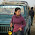can you please explain the steps

1.this is very easy concept and straight concept

2.Please post vhdl code for 8 bit wallace tree multiplier

3.This was really helpful!! :) Please post vhdl code wallace tree multipler for 8 bit...

4.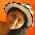Hi sir,
I am working on project "Multioperand Redundant Adders on FPGA" let me know how to write the code for this, plz give suggestion to me sir.

5.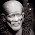Please post vhdl code wallace tree multipler for 8 bit...

6.For 8 bit codes or any other custom codes contact me by email. Note that I charge a fee for writing codes.

1.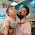Please send me ur email id

2.Your email id

3.kakdetushar1609@gmail.com

7.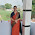please post vhdl code for modified booth encoder with wallace tree multiplier along with carry lookahead adder

8.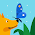would you please post code for 8bit wallace tree multiplier

1.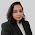use the above 4 bit code in structural model

9.Can you please tell me the logic of code given below for 4 bit wallace tree multiplier is correct or not.Because it is not working for some 4 bit combination such as (1011*0110 etc)

----------------------------------------------------------------------------------
-- Company:
-- Engineer:
--
-- Create Date: 21:51:25 02/16/2016
-- Design Name:
-- Module Name: wallace - Behavioral
-- Project Name:
-- Target Devices:
-- Tool versions:
-- Description:
--
-- Dependencies:
--
-- Revision:
-- Revision 0.01 - File Created
-- Additional Comments:
--
----------------------------------------------------------------------------------
library IEEE;
use IEEE.STD_LOGIC_1164.ALL;

-- Uncomment the following library declaration if using
-- arithmetic functions with Signed or Unsigned values
--use IEEE.NUMERIC_STD.ALL;

-- Uncomment the following library declaration if instantiating
-- any Xilinx primitives in this code.
--library UNISIM;
--use UNISIM.VComponents.all;

entity wallace is

Port ( A : in STD_LOGIC_VECTOR (3 downto 0);
B : in STD_LOGIC_VECTOR (3 downto 0);
P : out STD_LOGIC_VECTOR (7 downto 0));

end wallace;

architecture Behavioral of wallace is

component newand is
Port ( x : in STD_LOGIC;
y : in STD_LOGIC;
z : out STD_LOGIC);
end component;

component half_add is
Port ( p : in STD_LOGIC;
q : in STD_LOGIC;
Sum : out STD_LOGIC;
Carry : out STD_LOGIC);

end component;

component fullader_1 is
Port ( a : in STD_LOGIC;
b : in STD_LOGIC;
cin : in STD_LOGIC;
sum : out STD_LOGIC;
carry : out STD_LOGIC);
end component;

signal TEMP:std_logic_vector(14 downto 0);
signal s:STD_LOGIC_vector(3 downto 0);
signal Z:STD_LOGIC_vector(8 downto 0);

begin
and_2:newand port map(A(0),B(0),P(0));
and_3:newand port map(A(1),B(0),TEMP(0));
and_4:newand port map(A(0),B(1),TEMP(1));
and_5:newand port map(A(2),B(0),TEMP(2));
and_6:newand port map(A(1),B(1),TEMP(3));
and_7:newand port map(A(0),B(2),TEMP(4));
and_8:newand port map(A(3),B(0),TEMP(5));
and_9:newand port map(A(2),B(1),TEMP(6));
and_10:newand port map(A(1),B(2),TEMP(7));
and_11:newand port map(A(0),B(3),TEMP(8));
and_12:newand port map(A(3),B(1),TEMP(9));
and_13:newand port map(A(2),B(2),TEMP(10));
and_14:newand port map(A(1),B(3),TEMP(11));
and_15:newand port map(A(3),B(2),TEMP(12));
and_16:newand port map(A(2),B(3),TEMP(13));
and_17:newand port map(A(3),B(3),TEMP(14));

H:half_add port map(TEMP(0),TEMP(1),P(1),Z(0));
FULLADER1:fullader_1 port map(Z(0),TEMP(4),TEMP(3),s(0),Z(1));
FULLADER2:fullader_1 port map(s(0),Z(1),TEMP(2),P(2),Z(2));
FULLADER3:fullader_1 port map(Z(2),TEMP(8),TEMP(7),s(1),Z(3));
FULLADER4:fullader_1 port map(s(1),Z(3),TEMP(6),s(2),Z(4));
FULLADER5:fullader_1 port map(s(2),Z(4),TEMP(5),P(3),Z(5));
FULLADER6:fullader_1 port map(Z(5),TEMP(11),TEMP(10),s(3),Z(6));
FULLADER7:fullader_1 port map(s(3),Z(6),TEMP(9),P(4),Z(7));
FULLADER8:fullader_1 port map(Z(7),TEMP(13),TEMP(12),P(5),Z(8));

HALFADDER2:half_add port map(Z(8),TEMP(14),P(6),P(7));

end Behavioral;

10.we want code for 16 bit wallace tree mulptiplier

11.man, can you post code for 8 bit wallace tree multiplier